# Wave Walker DSP

## DSP Algorithms for RF Systems

DSP for Beginners: Simple Explanations for Complex Numbers! The second edition includes a new chapter on complex sinusoids.

Deriving the Ideal Low Pass Filter (LPF)
October 1, 2022

#### Introduction

This blog derives the ideal low pass filter (LPF) step by step using simple math.

More DSP blogs!

#### Frequency Domain Ideal Low Pass Filter

An ideal low pass filter (LPF) allows signals below the cutoff frequency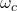to pass through unmodified with a linear gain of 1 and no phase change, while simultaneously completely rejecting all frequencies above the cutoff frequency with a linear gain of 0.

Mathematically, the ideal LPF is defined in the frequency domain by [oppenheim1999, p.43]

(1)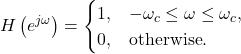The frequency response for the ideal LPF in (1) for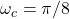is given in Figure 1.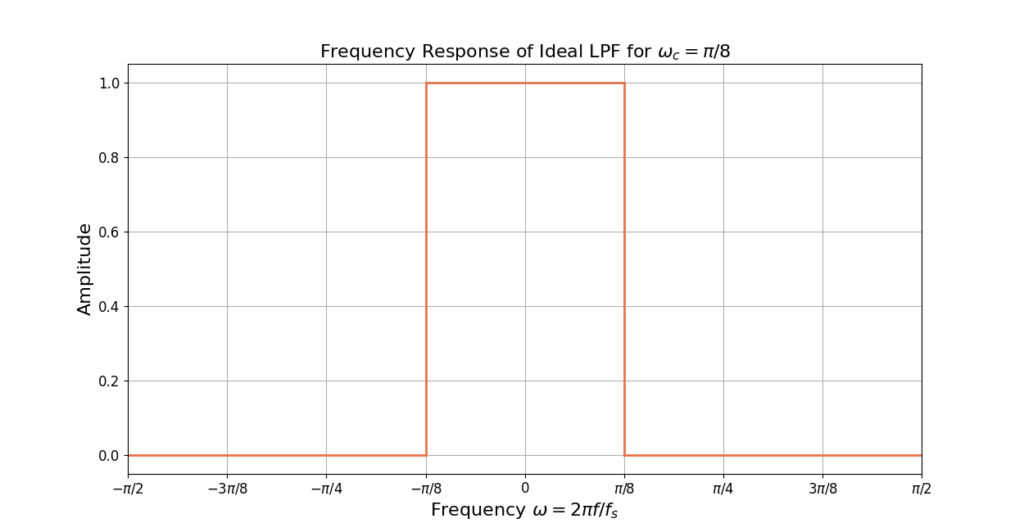Figure 1: The frequency response of the ideal low pass filter (LPF) for omega c = 1/8.

#### Time Domain Ideal Low Pass Filter

The ideal LPF in the time domain is derived by taking the inverse discrete-time Fourier transform (IDTFT) of (1),

(2)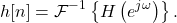The IDTFT is defined as

(3)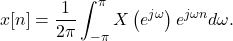Substituting (1) into (3),

(4)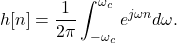The integral in (4) is simplified via Euler’s formula as

(5)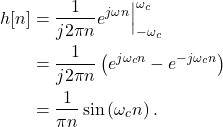The cutoff frequencyis the frequency in radians, defined as

(6)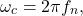where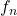is the frequency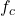in Hz normalized by the sampling rate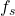,

(7)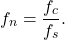The impulse response h[n] can therefore be written as

(8)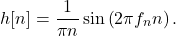Equation (8) can then be transformed into a sinc function where

(9)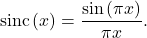The impulse response h[n] can therefore be written as

(10)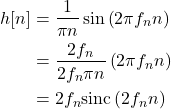The impulse response from (10) is given in Figure 2.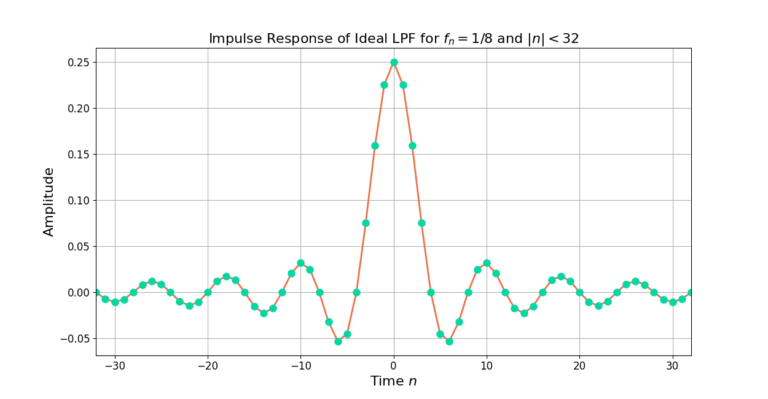Figure 2: The impulse response for the ideal low pass filter (LPF) for fn = 1/8.

#### Conclusion

The ideal low pass filter is a finite-length rectangle in the frequency domain but an infinitely long sinc in the time domain. Selecting the cutoff frequency determines which frequencies will be passed by the filter and which will be attenuated.

More DSP blogs!

God, the Lord, is my strength; he makes my feet like the deer’s; he makes me tread on my high places. Habakkuk 3:19

This website participates in the Amazon Associates program. As an Amazon Associate I earn from qualifying purchases.